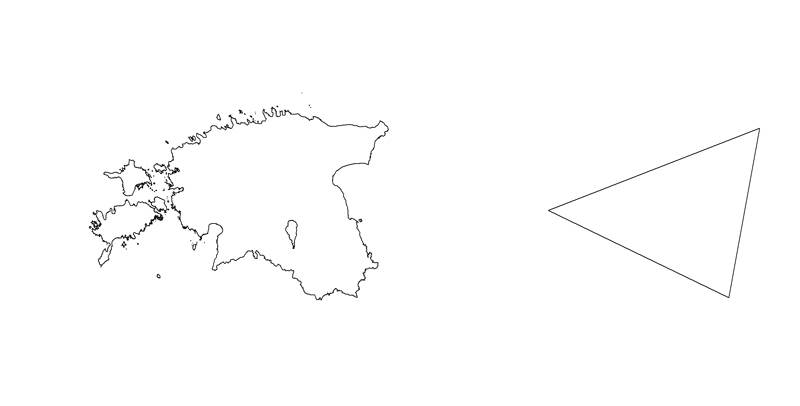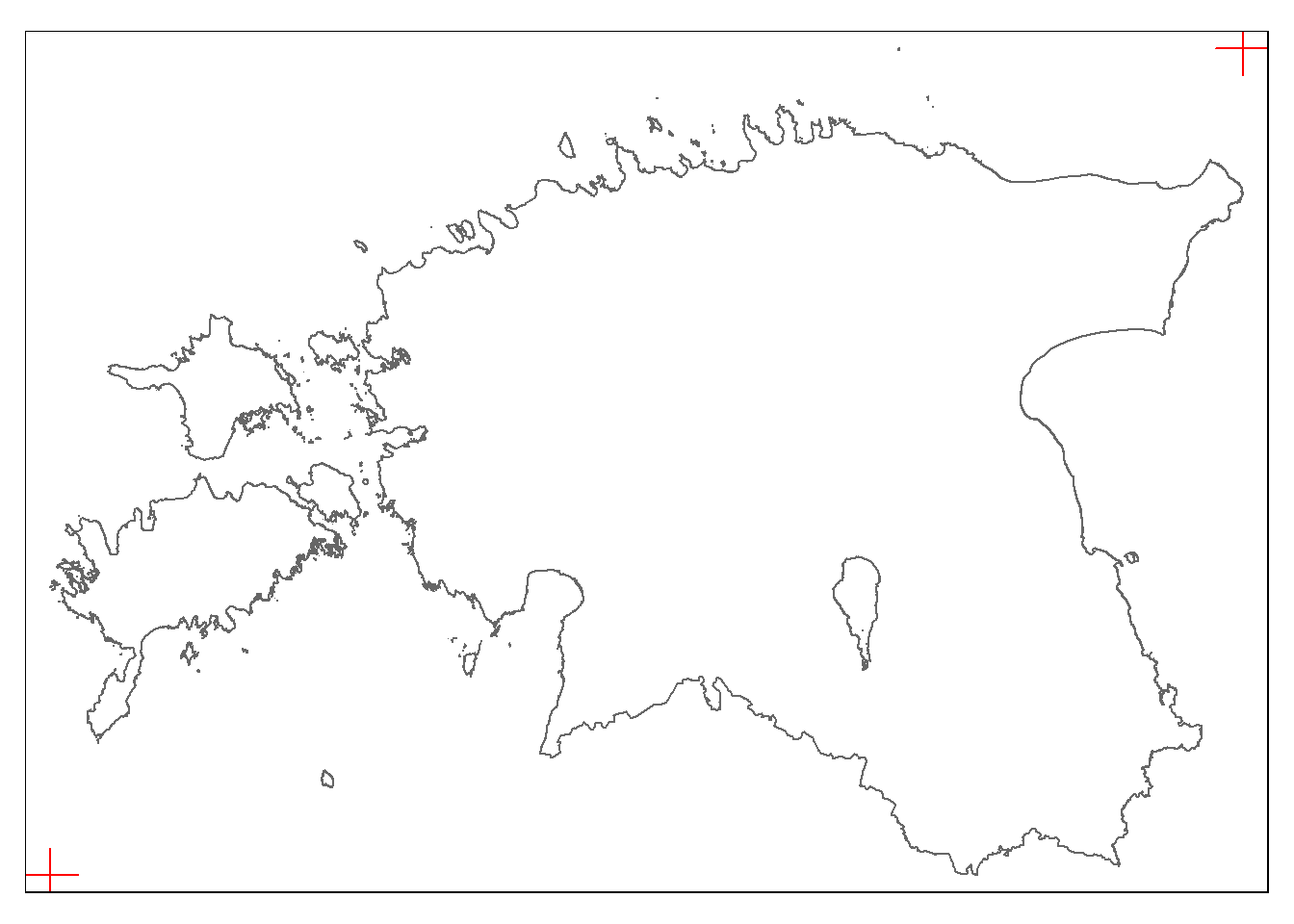libraries:

``````library(tidyverse)
library(ggthemes)
library(plotly)
library(sf)
library(leaflet)
library(gganimate)
library(tmap)``````

Animation is a method in which pictures are manipulated to appear as moving images (link). Basically it’s a sequence of images (rapid succession of sequential images).

Actually we already tried different animations during the previous practical sessions. But let’s look it now more closely. In general animations can be created for two purposes:

• process animation (changes in time),
• animation of static plot to reveal different angles.

## Animation as sequence of images

In next example we try to create the animation, where we demonstrate how the contour of Estonia is changing during the simplification process:``````download.file("http://aasa.ut.ee/GisMaps/data/est_wgs84.zip", destfile = "est_wgs84.zip")
unzip("est_wgs84.zip")

``````## Reading layer `eestimaa_wgs84' from data source
##   `C:\ANTO\loengud\geopythonR\rspatial_Git\rspatial\eestimaa_wgs84.shp'
##   using driver `ESRI Shapefile'
## Simple feature collection with 1 feature and 1 field
## Geometry type: MULTIPOLYGON
## Dimension:     XY
## Bounding box:  xmin: 21.76431 ymin: 57.50931 xmax: 28.209 ymax: 59.82202
## Geodetic CRS:  WGS 84``````
``````# transform it to 3301:
est_cont_3301 <- st_transform(est_cont, 3301)

# simplfy /generalize:
est_cont_3301_smpl <- st_simplify(est_cont_3301, preserveTopology = F, dTolerance = 200)``````

Now your task is to create sequence of images, where every next image is a bit more simplified than previous. First we create the vector of simplification level (dTolerance) for every step :

``````# create data frame, where first value of dTolerance is 102
steps <- data.frame(step = 102) # 102 comes after testinfg several different values

# Run the for/next loop to create the vector:
for(j in 1 : 100){
steps_tmp <- steps[j,] # take last dTolerance value
steps_tmp <- 1.07 * steps_tmp # multiply the value by 1.02 (+2%)
steps <- rbind(steps, data.frame(step = steps_tmp)) # bind the new value to data frame as new row
}``````

First 10 steps:

``head(steps, n = 10)``
``````##        step
## 1  102.0000
## 2  109.1400
## 3  116.7798
## 4  124.9544
## 5  133.7012
## 6  143.0603
## 7  153.0745
## 8  163.7897
## 9  175.2550
## 10 187.5228``````

Last 10 steps:

``tail(steps, n = 10)``
``````##         step
## 92  48141.98
## 93  51511.92
## 94  55117.75
## 95  58975.99
## 96  63104.31
## 97  67521.62
## 98  72248.13
## 99  77305.50
## 100 82716.88
## 101 88507.07``````

In the next step we create a fixed frame around Estonia. Otherwise, the animation will start “jumping around”. For that we use a bounding box which will be converted to a polygon:

``````a <- st_bbox(est_cont_3301_smpl)

# Result:
a``````
``````##      xmin      ymin      xmax      ymax
##  369047.8 6377146.4  739155.1 6634019.1``````
``````# convert it to dataframe:
a <- data.frame(x = c(a, a), y = c(a, a))
a``````
``````##             x       y
## xmin 369047.8 6377146
## xmax 739155.1 6634019``````
``````# sf object:
a <- st_as_sf(a, coords=c("x", "y"), crs = 3301)``````

Plot the bbox and contour of Estonia:

``tmap_mode("plot") # confirm the static mode``
``## tmap mode set to plotting``
``````tm_shape(a)+
tm_dots("red", size= 2, shape = 3)+
tm_shape(est_cont)+
tm_borders()``````To keep stuff in folder tidy, we create sub-directory for animation frames:

``dir.create("./anim")``

In next stage we produce animation frames. We are using the simple for/next loop, where simplified contour is created for every simplification step:

``````# create the image/map for every generalization step:
for(i in 1 : nrow(steps)){
est_cont_ee <- est_cont_3301 # create copy of contour
est_cont_ee <- st_simplify(est_cont_ee, dTolerance = steps[i,1]) # simplification for level i

# name of image (number in sequence, based on this numbering the images are bound together):

# previous step is needed because numbering in file name should be in format 0008, ,009, 0010 etc (not: 8, 9, 10 etc)!
myplot <- ggplot()+
theme_minimal()+
geom_sf(data = a, colour="white")+
geom_sf(data = est_cont_ee)

# save the frame
ggsave(filename = paste0("./anim/anim_", frameName, ".png"), plot = myplot, dpi = 100, width = 5, height = 4, units = "in")

# visualize the progress:
print(i)

}``````

Now you should have 101 images of Estonian contour.

Next stage assumes, that you have ffmpeg installed in your computer and it’s accessible from command line. Step-by-step tutorial How to install FFmpeg on Windows.
ffmpeg doesn’t have the graphical user interface (GUI). It can be used from terminal (e.g. Command Prompt), but you can run it directly from R as well:

``````#Terminal:
cd C:/ANTO/loengud/geopythonR/2019/rmd/anim/
ffmpeg -start_number 1 -i anim_%04d.png -vcodec libx264 -crf 25  -pix_fmt yuv420p test.mp4

#RStudio:
system("cd C:/ANTO/loengud/geopythonR/2019/rmd/anim/")
system("ffmpeg -start_number 1 -i anim_%04d.png -vcodec libx264 -crf 25  -pix_fmt yuv420p test.mp4")``````

FFmpeg is very powerful software. You can create animations, add soundtrack, titles etc. Unfortunately it’s not intuitive and first steps can be really hard. But if you mange, you can create something like that:

If ffmpeg is for the beginner a bit hard, then `magick` can help as well. Here is an example how to produce GIF:

``library(magick)``
``## Warning: package 'magick' was built under R version 4.1.1``
``````## Linking to ImageMagick 6.9.12.3
## Enabled features: cairo, freetype, fftw, ghostscript, heic, lcms, pango, raw, rsvg, webp
## Disabled features: fontconfig, x11``````
``````## list file names and read in
imgs <- list.files("./anim/", full.names = TRUE)